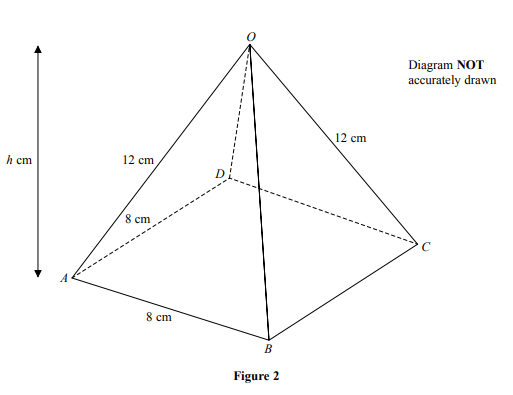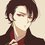# How to approach this questionLet midpoint of $$OA$$ be $$P$$ and $$Q$$ is the point on $$BC$$ such that $$BQ : QC = 3 : 1$$

Find the length of $PQ.$

Meanwhile $h = 4 \sqrt7$ cm

[ You are expected to use Pythagorean theorem only ]Note by Syed Hamza Khalid
1 year, 5 months ago

This discussion board is a place to discuss our Daily Challenges and the math and science related to those challenges. Explanations are more than just a solution — they should explain the steps and thinking strategies that you used to obtain the solution. Comments should further the discussion of math and science.

When posting on Brilliant:

• Use the emojis to react to an explanation, whether you're congratulating a job well done , or just really confused .
• Ask specific questions about the challenge or the steps in somebody's explanation. Well-posed questions can add a lot to the discussion, but posting "I don't understand!" doesn't help anyone.
• Try to contribute something new to the discussion, whether it is an extension, generalization or other idea related to the challenge.

MarkdownAppears as
*italics* or _italics_ italics
**bold** or __bold__ bold
- bulleted- list
• bulleted
• list
1. numbered2. list
1. numbered
2. list
Note: you must add a full line of space before and after lists for them to show up correctly
paragraph 1paragraph 2

paragraph 1

paragraph 2

[example link](https://brilliant.org)example link
> This is a quote
This is a quote
    # I indented these lines
# 4 spaces, and now they show
# up as a code block.

print "hello world"
# I indented these lines
# 4 spaces, and now they show
# up as a code block.

print "hello world"
MathAppears as
Remember to wrap math in $$ ... $$ or $ ... $ to ensure proper formatting.
2 \times 3 $2 \times 3$
2^{34} $2^{34}$
a_{i-1} $a_{i-1}$
\frac{2}{3} $\frac{2}{3}$
\sqrt{2} $\sqrt{2}$
\sum_{i=1}^3 $\sum_{i=1}^3$
\sin \theta $\sin \theta$
\boxed{123} $\boxed{123}$

Sort by:

Let the center of the base square $ABCD$ be the origin $(0,0,0)$ and $A(4,-4,0)$, $B(4,4,0)$, $C(-4,4,0)$, $D(-4,-4,0)$, and $O(0,0,h) = O(0,0,4\sqrt 7)$. Note that $h = \sqrt{12^2-(4\sqrt 2)^2} = 4\sqrt 7$.

Then $P \left(\frac {4+0}2, \frac {-4+0}2, \frac {0+4\sqrt 7}2\right) = P (2,-2,2\sqrt 7)$ and $Q(-2,4,0)$. By Pythagorean theorem:

\begin{aligned} PQ^2 & = (2-(-2))^2 + (-2-4)^2 + (2\sqrt 7-0)^2 = 80 \\ \implies PQ & = \boxed{4\sqrt 5} \end{aligned}

- 1 year, 5 months ago

Nop. PQ = $4 \sqrt{5}$ but I don't know how

- 1 year, 5 months ago

Note that $h$ cannot be $\text{12 cm}$ because the slanting sides $AO=BO=CO=DO=\text{12 cm}$.

- 1 year, 5 months ago

I have got it. $h = 4\sqrt 7$.

- 1 year, 5 months ago

Yea I am sorry it is just as you said. I have edited the problem

- 1 year, 5 months ago

But still the ans for PQ is $4 \sqrt{5}$ how?

- 1 year, 5 months ago

on point!

- 1 year, 5 months ago

the problem says that h (*height of the pyramid) = 12 and also OA = OD = OC = OB =12 , which is geometrically not possible.

- 1 year, 5 months ago

Yep. I'm sorry I mistyped.

- 1 year, 5 months ago

yep I will post the solution with diagram .. but it's not possible until you post this note as a problem

- 1 year, 5 months ago

The information $h=4\sqrt 7$ is not necessary for solving the problem. It can be found from other information given. It should be mentioned if it is set as a problem.

- 1 year, 5 months ago

you are absolutely right sir, do check my solution....here" https://brilliant.org/problems/i-dont-know-why-its-not-easy/#!/solution-comments/241234/"

- 1 year, 5 months ago

- 1 year, 5 months ago

I have provided the solution in the discussion panel of your problem named " I don't know why its not easy..." geometry level 2

- 1 year, 5 months ago

Oh okay... I checked its great. I clearly understand. Thank you so much for solving it.

- 1 year, 5 months ago

not mention, it's my pleasure

- 1 year, 5 months ago